Asset Pricing

In the previous sections we used the Euler equation to derive optimal consumption and investment decisions. Now note that the Euler equation defines a relation between consumption (or other real variables) and prices.242

Previously we took the prices as given and derived the optimal path of consumption. We may, however, use the Euler relation in the other direction. Take the path of real variables, e.g., consumption as given and derive what the prices have to be. A straightforward way to do this is to assume that output is exogenous, like manna from heaven, and cannot be stored. In that environment we may introduce markets for capital and production facilities. This is the setup in the seminal Lucas (Econometrica, 1978) paper.

We will later relax the assumption about exogenous and non-storable output. This will give us the basic stochastic growth (or RBC) model.

Asset Pricing in the Lucas Tree Model and other CAPM

Asset Pricing in the Lucas Tree Model

Large number of identical agents.

Equal number of trees with stochastic crop dt The distribution of dt is Markov. Distribution is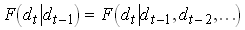. The process known by all agents.

Purpose: find pt – the price of a tree as a function of the state of the economy (dt).

The gross return on a tree is(per capita)

No safe asset.

Perfect market in ownership of trees. All equal so no trade in equilibrium.

No storage or foreign trade so consumption ct = dt

Substitute forfrom the Euler equation noting that w =0 gives243

By 7)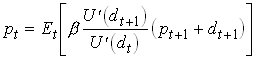For any type of expectations in based on dt we can compute a price today as a function of dt Let the individuals subjective expectations of dt+1 be described byand the expectations about the relation between pt+1 and dt+1 be given by the function ps(dt+1). We then have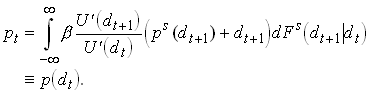Now Lucas defined the very powerful concept of rational expectations. Let’s require thatand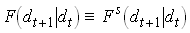. Lucas proves that this together with defines a unique and constant pricing function.

Use recursions onA discounted sum of dividends. Stochastic discount rates unless marginal utility is constant.

A simple example with log utility247

With dt i.i.d. so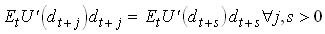248

Note that the price increases in dt (if U is concave).

Assume an autocorrelation in dt thendepends on dt Both income and substitution effect, with log utility they cancel.

The Consumption and market CAPM

Previously we found that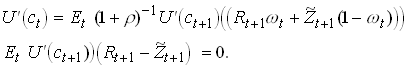The second equation in implies.

250

Substituting into the first equation in yields251

Consider a similar problem but with n risky assets each with a stochastic net return of(º-1). FOC for each risk asset i yields after the same substitution as aboveSoThis is the Consumption CAPM.

So a risky asset can have an expected rate of return that is larger or smaller than the safe return. Note that a positive covariance between consumption and the risky return implies a negative covariance between marginal utility and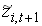so that asset will have a risk premium.

Note that if we have CRRA (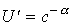) then we havewhere I have used that.

Now assume there exists an asset m which return is perfectly negatively correlated with marginal utility. So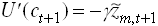and thus.

Then from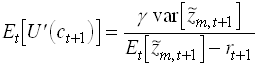Substitute intoThis is the market or traditional CAPM. Note that bi is the (true) regression coefficient in a regression of asset i on m. The term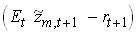can be interpreted as the price of aggregate or systematic risk.

Empirics

The Mehra – Prescott Puzzle

Consider a representative household that "maximizes"258

l is a stochastic growth rate that can take n different valuesall >0. The probability of a specific growth rate depends only on last periods growth rate..

259

Assume there is a share that entitles the owner to the entire output the next period. From we have that the price of this share is260

where i Î {1,...,n}. So the price is H(1) in c and y.

We can also use to get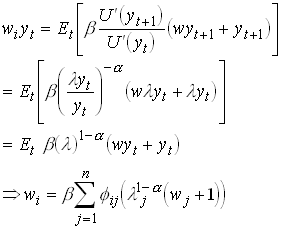261

This is a linear equation system in n unknowns so we can solve it for the price of the share in all states of the world. Now we can calculate the net return on the asset262

and expected return is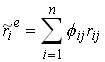263

We can also compute the price of a safe asset in this economy.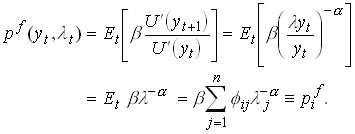264

with a return of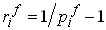.

Now we want to find the unconditional (average) returns on the assets. First we need the unconditional probabilities of the states p.

Assume ergodic growth rates265

Then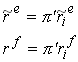266

The risk premium is then defined as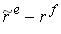.

Results

Now simplify and assumed there is two states and a common transition probability.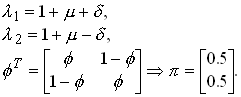267

Now we calibrate the model using the mean growth rate of GDP, its variance and autocorrelation.268

Using these value and some reasonable values for b and a <10 we find that the risk premium should be something in the order of 0 to 0.4%. But on average the US stock market has yielded 6% more on average than government.

Consumption versus market b (Mankiw & Shapiro 1986)

Mankiw and Shapiro first estimates from 464 different stocks. They first estimate the market b, i.e.,assuming it is constant over time. Note that the coefficient on b should be equal to the equity premium (around 6%) and constant for all periods and assets. Then they use this variable to predict the return on the corresponding asset.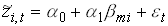269

The coefficient is significant and around 6 for most estimation methods.

Now rewrite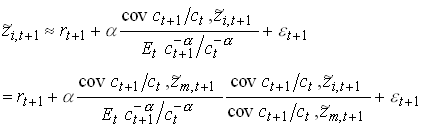Assume that all relevant moments in are constant over time. We then bci as sample moments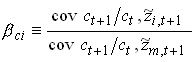271

Then we can run the regression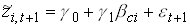272

Now the estimate of g1 is insignificant and unstable. M&S also run a regression with both b,  then only the coefficient on bmi comes out significant.